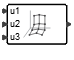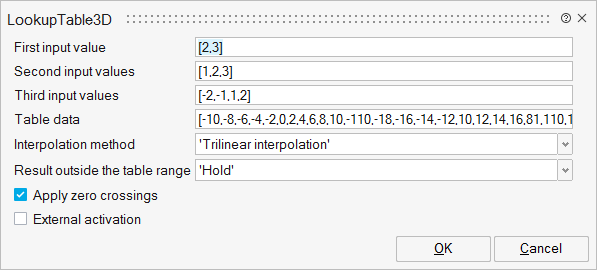# LookupTable3D

This block approximates a non-linear function using interpolation on data provided as a "3D-table".LookupTables

## Description

The LookupTable3D block approximates a non-linear function using interpolation on data provided as a "3D-table". The three-dimensional table is then defined by specifying three vectors (x, y, z) of size m, n, and k, and a "3D-table", a vector of size m*n*k. Output of the block is the approximation of the block input using the bilinear interpolation method.

Each input can be either scalar, vector or matrix.

## ParametersNameLabelDescriptionData TypeValid Values

xx

First input value

Vector of length m.

Vector

yy

Second input values

Vector of length n.

Vector

zz

Third input values

Vector of length k.

Vector

ff

Table data

vector of size (m x n x k).

Vector

Method

Interpolation method

String

'Trilinear
interpolation'
'Nearest_point'
'Point_just_below'
'Point_just_above'

DoOutside

Result outside the table range

String

'Zero'
'Hold'
'Trilinear
extrapolation'

EventGen

Apply zero crossings

Scalar

0
1

externalActivation

External activation

Specifies whether the block receives an external activation or inherits its activation through its regular input ports. When External Activation is selected, an additional activation port is added to the block. By default, external activation is not selected.

Number

0
1

## Ports

NameTypeDescriptionIO TypeNumber

Port 1

explicit

output

1

u1

explicit

input

1

u2

explicit

input

2

u3

explicit

input

3

Port 5

activation

input

externalActivation